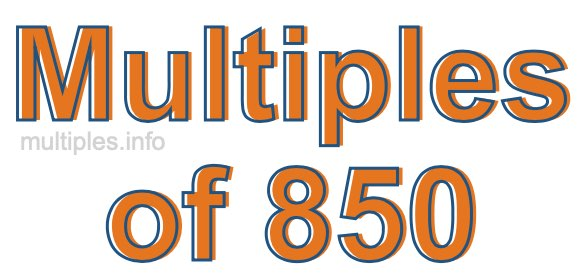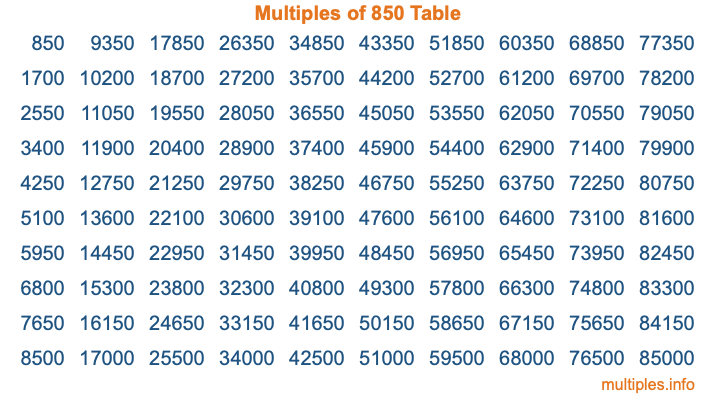Multiples of 850Welcome to the Multiples of 850 page. Here we will first teach you everything you will ever need to know about the multiples of 850, and then give you a study guide summary of everything we taught you to make sure you remember it all. Use this page to look up facts and learn information about the multiples of 850. This page will make you a multiples of eight hundred fifty expert!

Definition of Multiples of 850
Multiples of 850 are all the numbers that when divided by 850 equal an integer. Each of the multiples of 850 are called a multiple. A multiple of 850 is created by multiplying 850 by an integer.

Therefore, to create a list of multiples of 850, you start with 1 multiplied by 850, then 2 multiplied by 850, then 3 multiplied by 850, and so on for as long as you want. Thus, the list of the first five multiples of 850 is 850, 1700, 2550, 3400, and 4250. To see a larger list of multiples of 850, see the printable image of Multiples of 850 further down on this page. We also have a category where you can choose any nth multiple of 850.

Multiples of 850 Checker
The Multiples of 850 Checker below checks to see if any number of your choice is a multiple of 850. In other words, it checks to see if there is any number (integer) that when multiplied by 850 will equal your number. To do that, we divide your number by 850. If the the quotient is an integer, then your number is a multiple of 850.

Is  a multiple of 850?

Least Common Multiple of 850 and ...
A Least Common Multiple (LCM) is the lowest multiple that two or more numbers have in common. This is also called the smallest common multiple or lowest common multiple and is useful to know when you are adding our subtracting fractions. Enter one or more numbers below (850 is already entered) to find the LCM.

Check out our LCM Calculator if you need more details about the Least Common Multiple or if you need the LCM for different numbers for adding and subtraction fractions.

nth Multiple of 850
As we stated above, 850 is the first multiple of 850, 1700 is the second multiple of 850, 2550 is the third multiple of 850, and so on. Enter a number below to find the nth multiple of 850.

th multiple of 850

Multiples of 850 vs Factors of 850
850 is a multiple of 850 and a factor of 850, but that is where the similarities end. All postive multiples of 850 are 850 or greater than 850. All positive factors of 850 are 850 or less than 850.

Below is the beginning list of multiples of 850 and the factors of 850 so you can compare:

Multiples of 850: 850, 1700, 2550, 3400, 4250, etc.

Factors of 850: 1, 2, 5, 10, 17, 25, 34, 50, 85, 170, 425, 850

As you can see, the multiples of 850 are all the numbers that you can divide by 850 to get a whole number. The factors of 850, on the other hand, are all the whole numbers that you can multiply by another whole number to get 850.

It's also interesting to note that if a number (x) is a factor of 850, then 850 will also be a multiple of that number (x).

Multiples of 850 vs Divisors of 850
The divisors of 850 are all the integers that 850 can be divided by evenly. Below is a list of the divisors of 850.

Divisors of 850: 1, 2, 5, 10, 17, 25, 34, 50, 85, 170, 425, 850

The interesting thing to note here is that if you take any multiple of 850 and divide it by a divisor of 850, you will see that the quotient is an integer.

Multiples of 850 Table
Below is an image of the first 100 multiples of 850 in a table. The table is in chronological order, column by column. The first column has the first ten multiples of 850, the second column has the next ten multiples of 850, and so on.The Multiples of 850 Table is also referred to as the 850 Times Table or Times Table of 850. You are welcome to print out our table for your studies.

Negative Multiples of 850
Although not often discussed or needed in math, it is worth mentioning that you can make a list of negative multiples of 850 by multiplying 850 by -1, then by -2, then by -3, and so on, to get the following list of negative multiples of 850:

-850, -1700, -2550, -3400, -4250, etc.

Multiples of 850 Summary
Below is a summary of important Multiples of 850 facts that we have discussed on this page. To retain the knowledge on this page, we recommend that you read through the summary and explain to yourself or a study partner why they hold true.

There are an infinite number of multiples of 850.

A multiple of 850 divided by 850 will equal a whole number.

850 divided by a factor of 850 equals a divisor of 850.

The nth multiple of 850 is n times 850.

The largest factor of 850 is equal to the first positive multiple of 850.

850 is a multiple of every factor of 850.

850 is a multiple of 850.

A multiple of 850 divided by a divisor of 850 equals an integer.

850 divided by a divisor of 850 equals a factor of 850.

Any integer times 850 will equal a multiple of 850.

Multiples of a Number
Here you can get the multiples of another number, all with the same attention to detail as we did for multiples of 850 on this page.

Multiples of
Multiples of 851
Did you find our page about multiples of eight hundred fifty educational? Do you want more knowledge? Check out the multiples of the next number on our list!